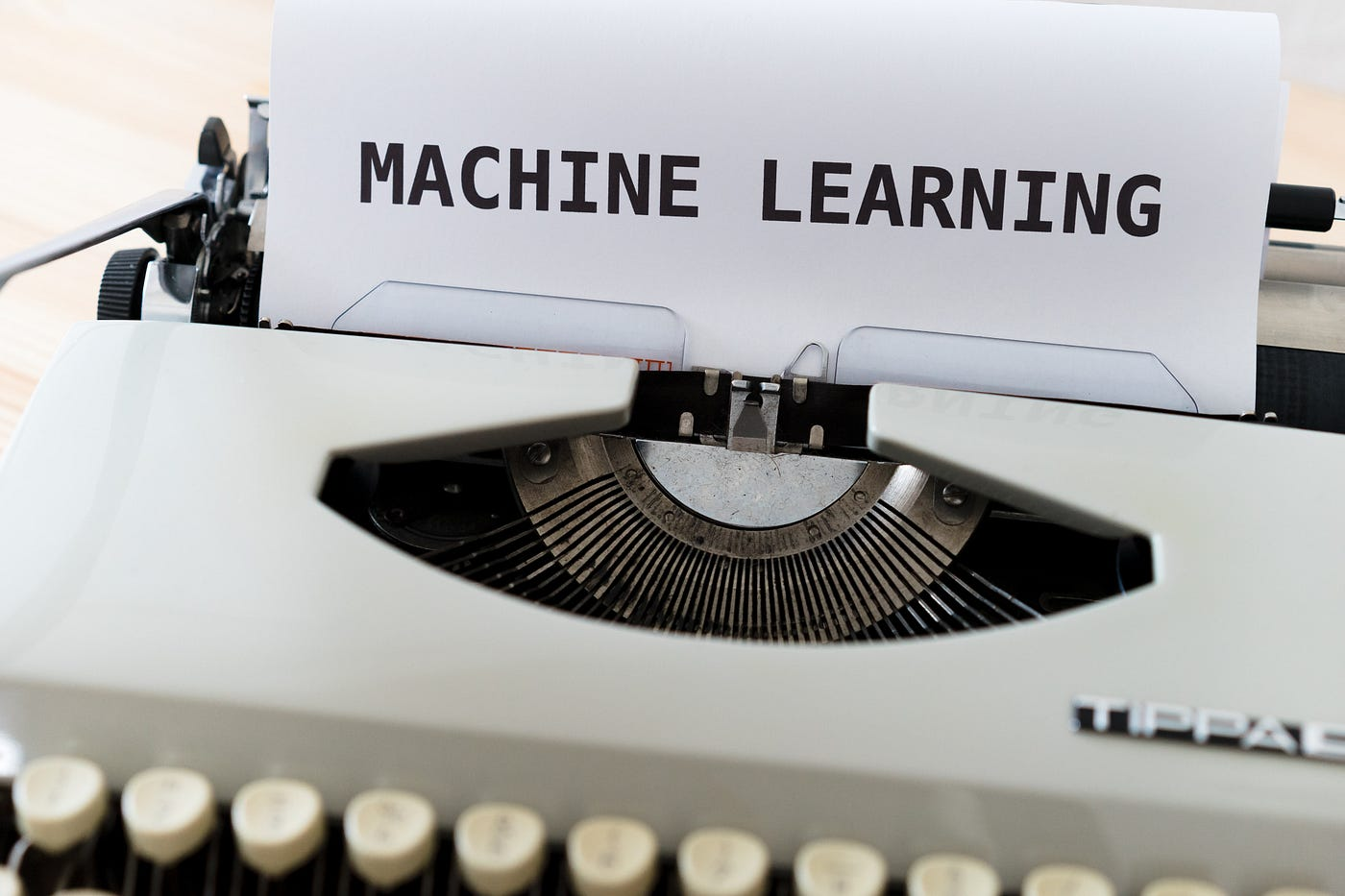< Back to Blog Overview

# Web Scraping & Data Preprocessing for a Machine Learning model

2020-07-03# Gathering the Data

## Setup

```mkdir scraper
pip install beautifulsoup4
pip install requests
pip install pandas```
```from bs4 import BeautifulSoup
import requests
import pandas as pd```

## Preparing the Food

```r = requests.get(‘https://milindjagre.co/2018/03/10/post-3-ml-data-preprocessing-part-1/’).textsoup=BeautifulSoup(r,'html.parser')u=list()
l={}```
`table = soup.find(“table”,{“class”:”js-csv-data csv-data js-file-line-container”}) tr = table.find_all(“tr”,{“class”:”js-file-line”})`
```for i in range(0,len(tr)):
td=tr[i].find_all(“td”)
for x in range(0,len(td)):
try:
l[“Country”]=td.text
except:
l[“Country”]=None  try:
l[“Age”]=td.text
except:
l[“Age”]=None  try:
l[“Salary”]=td.text
except:
l[“Salary”]=None  try:
l[“Purchased”]=td.text
except:
l[“Purchased”]=None  u.append(l)
l={}```
```{
“Data”: [
{
“Country”: “France”,
“Age”: “44”,
“Purchased”: “No”,
“Salary”: “72000”
},
{
“Country”: “France”,
“Age”: “44”,
“Purchased”: “No”,
“Salary”: “72000”
},
{
“Country”: “France”,
“Age”: “44”,
“Purchased”: “No”,
“Salary”: “72000”
},
{
“Country”: “France”,
“Age”: “44”,
“Purchased”: “No”,
“Salary”: “72000”
},
{
“Country”: “France”,
“Age”: “44”,
“Purchased”: “No”,
“Salary”: “72000”
},
{
“Country”: “Spain”,
“Age”: “27”,
“Purchased”: “Yes”,
“Salary”: “48000”
},
{
“Country”: “Spain”,
“Age”: “27”,
“Purchased”: “Yes”,
“Salary”: “48000”
},
{
“Country”: “Spain”,
“Age”: “27”,
“Purchased”: “Yes”,
“Salary”: “48000”
},
{
“Country”: “Spain”,
“Age”: “27”,
“Purchased”: “Yes”,
“Salary”: “48000”
},
{
“Country”: “Spain”,
“Age”: “27”,
“Purchased”: “Yes”,
“Salary”: “48000”
},
{
“Country”: “Germany”,
“Age”: “30”,
“Purchased”: “No”,
“Salary”: “54000”
},
{
“Country”: “Germany”,
“Age”: “30”,
“Purchased”: “No”,
“Salary”: “54000”
},
{
“Country”: “Germany”,
“Age”: “30”,
“Purchased”: “No”,
“Salary”: “54000”
},
{
“Country”: “France”,
“Age”: “37”,
“Purchased”: “Yes”,
“Salary”: “67000”
}
]
}```
```df = pd.io.json.json_normalize(u)
df.to_csv(‘data.csv’, index=False, encoding=’utf-8')```

# Import the data and libraries

`datasets = pd.read_csv(‘Data.csv’)`

# Dependent & Independent Variables

`X = datasets.iloc[: , :-1].values`
`y = datasets.iloc[: , 3].values`

# Missing values

```from sklearn.impute import SimpleImputer
imputer = SimpleImputer(missing_values=np.nan,strategy=’mean’)
imputer = imputer.fit(X[:, 1:3])
X[:,1:3] = imputer.transform(X[:,1:3])```
```[[‘France’ 44.0 72000.0]
[‘Spain’ 27.0 48000.0]
[‘Germany’ 30.0 54000.0]
[‘Spain’ 38.0 61000.0]
[‘Germany’ 40.0 63777.77777777778]
[‘France’ 35.0 58000.0]
[‘Spain’ 38.77777777777778 52000.0]
[‘France’ 48.0 79000.0]
[‘Germany’ 50.0 83000.0]
[‘France’ 37.0 67000.0]]```

# Categorical variables

```from sklearn.preprocessing import LabelEncoder
labelencoder_X = LabelEncoder()
X[:,0] = labelencoder_X.fit_transform(X[:,0])```
```[[0 44.0 72000.0]
[2 27.0 48000.0]
[1 30.0 54000.0]
[2 38.0 61000.0]
[1 40.0 63777.77777777778]
[0 35.0 58000.0]
[2 38.77777777777778 52000.0]
[0 48.0 79000.0]
[1 50.0 83000.0]
[0 37.0 67000.0]]```

## Dummy Variable

```from sklearn.compose import ColumnTransformer
from sklearn.preprocessing import OneHotEncoder
ct = ColumnTransformer([(“Country”, OneHotEncoder(), )], remainder = ‘passthrough’)
X = ct.fit_transform(X)```
```[[1.0 0.0 0.0 44.0 72000.0]
[0.0 0.0 1.0 27.0 48000.0]
[0.0 1.0 0.0 30.0 54000.0]
[0.0 0.0 1.0 38.0 61000.0]
[0.0 1.0 0.0 40.0 63777.77777777778]
[1.0 0.0 0.0 35.0 58000.0]
[0.0 0.0 1.0 38.77777777777778 52000.0]
[1.0 0.0 0.0 48.0 79000.0]
[0.0 1.0 0.0 50.0 83000.0]
[1.0 0.0 0.0 37.0 67000.0]]```

## Independent Variable

```labelencoder_y = LabelEncoder()
y = labelencoder_y.fit_transform(y)```
`[0 1 0 0 1 1 0 1 0 1]`

# Split the dataset into training and test set

```from sklearn.model_selection import train_test_split
X_train, X_test, y_train, y_test = train_test_split(X, y, test_size=0.2, random_state=42)```

# Feature Scaling

```from sklearn.preprocessing import StandardScaler
sc_X = StandardScaler()
X_train = sc_X.fit_transform(X_train)
X_test = sc_X.transform(X_test)```

# Conclusion